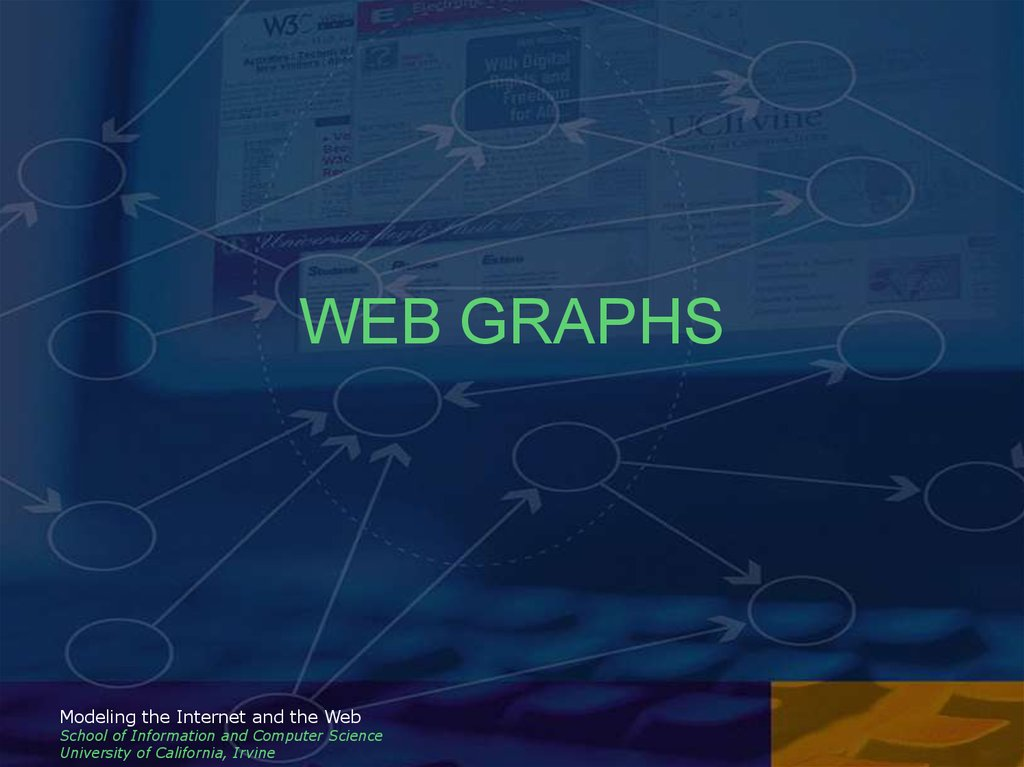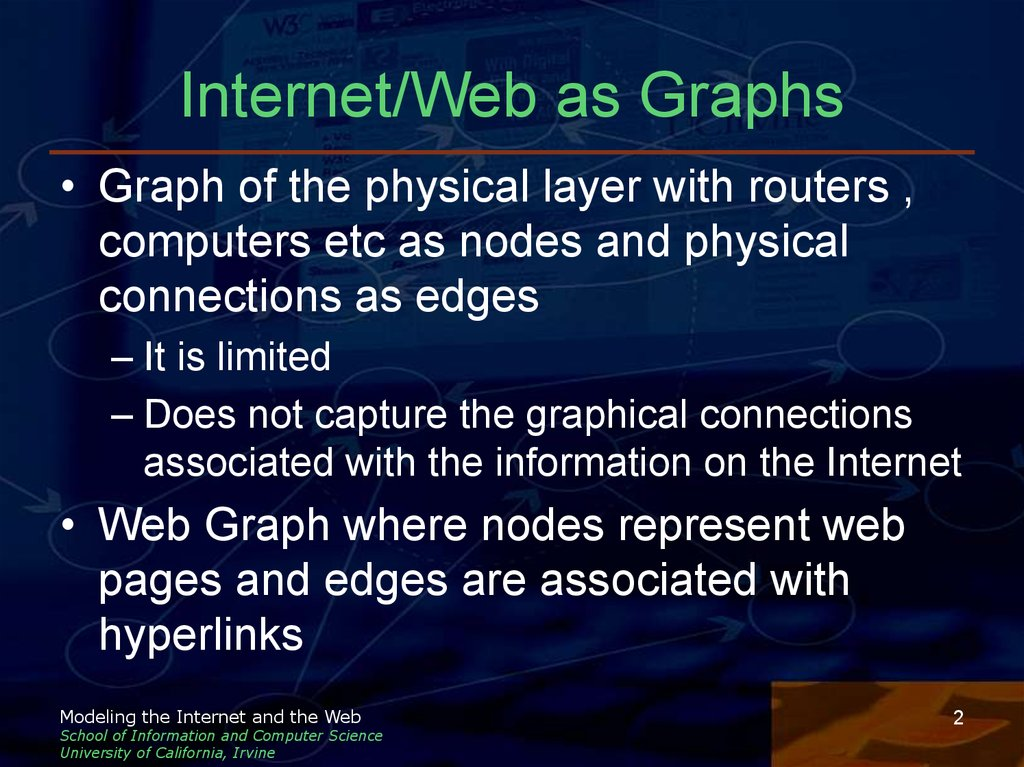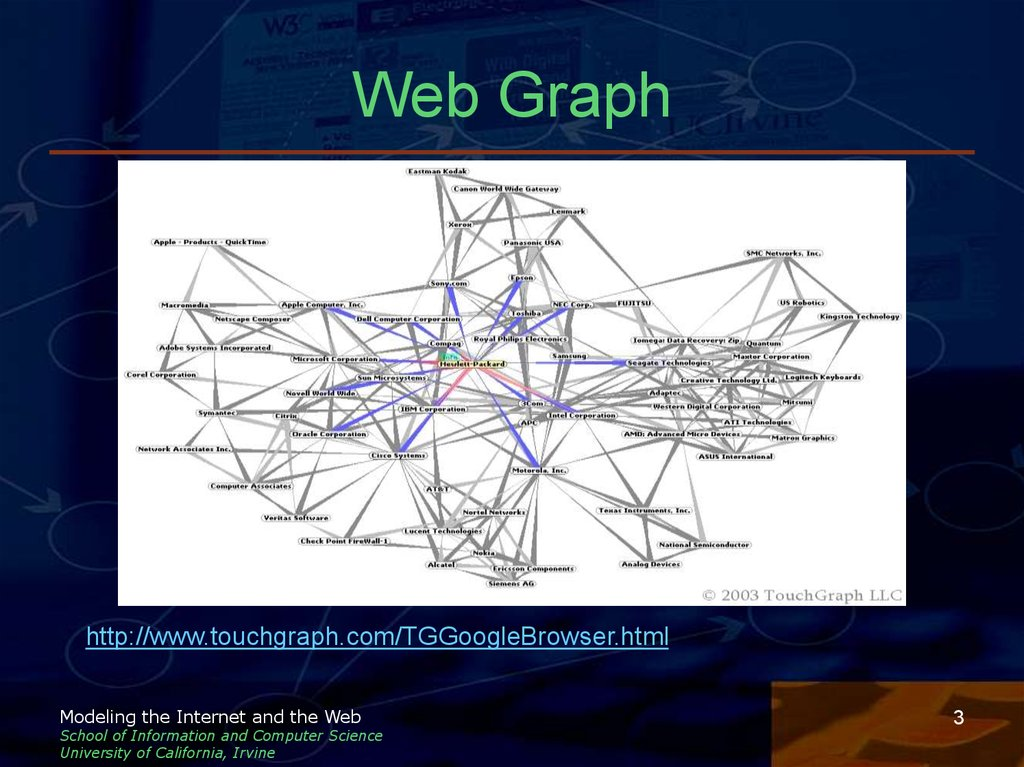# WEB GRAPHS/ Modeling the Internet and the Web School of Information and Computer Science

## 1. WEB GRAPHS

Modeling the Internet and the Web
School of Information and Computer Science
University of California, Irvine

## 2. Internet/Web as Graphs

• Graph of the physical layer with routers ,
computers etc as nodes and physical
connections as edges
– It is limited
– Does not capture the graphical connections
associated with the information on the Internet
• Web Graph where nodes represent web
pages and edges are associated with
Modeling the Internet and the Web
School of Information and Computer Science
University of California, Irvine
2

## 3. Web Graph

Modeling the Internet and the Web
School of Information and Computer Science
University of California, Irvine
3

## 4. Web Graph Considerations

• Edges can be directed or undirected
• Graph is highly dynamic
– Nodes and edges are added/deleted often
– Content of existing nodes is also subject to
change
– Pages and hyperlinks created on the fly
• Apart from primary connected component
there are also smaller disconnected
components
Modeling the Internet and the Web
School of Information and Computer Science
University of California, Irvine
4

## 5. Why the Web Graph?

• Example of a large,dynamic and
distributed graph
• Possibly similar to other complex graphs in
social, biological and other systems
• Reflects how humans organize information
(relevance, ranking) and their societies
• Study behavior of users as they traverse
the web graph (e-commerce)
Modeling the Internet and the Web
School of Information and Computer Science
University of California, Irvine
5

## 6. Statistics of Interest

Size and connectivity of the graph
Number of connected components
Distribution of pages per site
Distribution of incoming and outgoing
connections per site
• Average and maximal length of the
shortest path between any two vertices
(diameter)
Modeling the Internet and the Web
School of Information and Computer Science
University of California, Irvine
6

## 7. Properties of Web Graphs

• Connectivity follows a power law
distribution
• The graph is sparse
– |E| = O(n) or atleast o(n2)
– Average number of hyperlinks per page
roughly a constant
• A small world graph
Modeling the Internet and the Web
School of Information and Computer Science
University of California, Irvine
7

## 8. Power Law Size

• Simple estimates suggest over a billion
nodes
• Distribution of site sizes measured by the
number of pages follow a power law
distribution
• Observed over several orders of
magnitude with an exponent g in the 1.61.9 range
Modeling the Internet and the Web
School of Information and Computer Science
University of California, Irvine
8

## 9. Power Law Connectivity

• Distribution of number of connections per
node follows a power law distribution
• Study at Notre Dame University reported
– g = 2.45 for outdegree distribution
– g = 2.1 for indegree distribution
• Random graphs have Poisson distribution
if p is large.
– Decays exponentially fast to 0 as k increases
towards its maximum value n-1
Modeling the Internet and the Web
School of Information and Computer Science
University of California, Irvine
9

## 10. Power Law Distribution -Examples

Power Law Distribution Examples
http://www.pnas.org/cgi/reprint/99/8/5207.pdf
Modeling the Internet and the Web
School of Information and Computer Science
University of California, Irvine
10

## 11. Examples of networks with Power Law Distribution

Internet at the router and interdomain level
Citation network
Collaboration network of actors
Networks associated with metabolic
pathways
• Networks formed by interacting genes and
proteins
• Network of nervous system connection in
C. elegans
Modeling the Internet and the Web
School of Information and Computer Science
University of California, Irvine
11

## 12. Small World Networks

• It is a ‘small world’
– Millions of people. Yet, separated by “six
degrees” of acquaintance relationships
– Popularized by Milgram’s famous experiment
• Mathematically
– Diameter of graph is small (log N) as
compared to overall size
• 3. Property seems interesting given ‘sparse’ nature
of graph but …
• This property is ‘natural’ in ‘pure’ random graphs
Modeling the Internet and the Web
School of Information and Computer Science
University of California, Irvine
12

## 13. The small world of WWW

• Empirical study of Web-graph reveals
small-world property
– Average distance (d) in simulated web:
e.g.
d = 0.35 + 2.06 log (n)
n = 109, d ~= 19
– Graph generated using power-law model
– Diameter properties inferred from sampling
• Calculation of max. diameter computationally
demanding for large values of n
Modeling the Internet and the Web
School of Information and Computer Science
University of California, Irvine
13

## 14. Implications for Web

• Logarithmic scaling of diameter makes
future growth of web manageable
– 10-fold increase of web pages results in only
2 more additional ‘clicks’, but …
– Users may not take shortest path, may use
bookmarks or just get distracted on the way
– Therefore search engines play a crucial role
Modeling the Internet and the Web
School of Information and Computer Science
University of California, Irvine
14

## 15. Some theoretical considerations

• Classes of small-world networks
– Scale-free: Power-law distribution of connectivity
over entire range
abrupt cut-off
– Single-scale: Connectivity distribution decays
exponentially
Modeling the Internet and the Web
School of Information and Computer Science
University of California, Irvine
15

## 16. Power Law of PageRank

• Assess importance of a page relative to a
query and rank pages accordingly
– Importance measured by indegree
– Not reliable since it is entirely local
• PageRank – proportion of time a random
surfer would spend on that page at steady
state
• A random first order Markov surfer at each
time step travels from one page to another
Modeling the Internet and the Web
School of Information and Computer Science
University of California, Irvine
16

## 17. PageRank contd

• Page rank r(v) of page v is the steady
state distribution obtained by solving the
system of linear equations given by
Where pa[v] = set of parent nodes
Ch[u] = out degree
Modeling the Internet and the Web
School of Information and Computer Science
University of California, Irvine
17

## 18. Examples

• Log Plot of PageRank Distribution of Brown Domain
(*.brown.edu)
G.Pandurangan, P.Raghavan,E.Upfal,”Using PageRank to characterize Webstructure”
,COCOON 2002
Modeling the Internet and the Web
School of Information and Computer Science
University of California, Irvine
18

## 19. Bow-tie Structure of Web

• A large scale study (Altavista crawls)
reveals interesting properties of web
– Study of 200 million nodes & 1.5 billion links
– Small-world property not applicable to entire
web
• Some parts unreachable
• Others have long paths
– Power-law connectivity holds though
• Page indegree (g = 2.1), outdegree (g = 2.72)
Modeling the Internet and the Web
School of Information and Computer Science
University of California, Irvine
19

## 20. Bow-tie Components

• Strongly Connected
Component (SCC)
– Core with small-world
property
• Upstream (IN)
– Core can’t reach IN
• Downstream (OUT)
– OUT can’t reach core
• Disconnected (Tendrils)
Modeling the Internet and the Web
School of Information and Computer Science
University of California, Irvine
20

## 21. Component Properties

• Each component is roughly same size
– ~50 million nodes
• Tendrils not connected to SCC
– But reachable from IN and can reach OUT
• Tubes: directed paths IN->Tendrils->OUT
• Disconnected components
– Maximal and average diameter is infinite
Modeling the Internet and the Web
School of Information and Computer Science
University of California, Irvine
21

## 22. Empirical Numbers for Bow-tie

• Maximal minimal (?) diameter
– 28 for SCC, 500 for entire graph
• Probability of a path between any 2 nodes
– ~1 quarter (0.24)
• Average length
– 16 (directed path exists), 7 (undirected)
• Shortest directed path between 2 nodes in
Modeling the Internet and the Web
School of Information and Computer Science
University of California, Irvine
22

## 23. Models for the Web Graph

• Stochastic models that can explain or
atleast partially reproduce properties of the
web graph
– The model should follow the power law
distribution properties
– Represent the connectivity of the web
– Maintain the small world property
Modeling the Internet and the Web
School of Information and Computer Science
University of California, Irvine
23

## 24. Web Page Growth

• Empirical studies observe a power law
distribution of site sizes
– Size includes size of the Web, number of IP
addresses, number of servers, average size
of a page etc
• A Generative model is being proposed to
account for this distribution
Modeling the Internet and the Web
School of Information and Computer Science
University of California, Irvine
24

## 25. Component One of the Generative Model

• The first component of this model is that
“ sites have short-term size fluctuations up or
down that are proportional to the size of the
site “
• A site with 100,000 pages may gain or
lose a few hundred pages in a day
whereas the effect is rare for a site with
only 100 pages
Modeling the Internet and the Web
School of Information and Computer Science
University of California, Irvine
25

## 26. Component Two of the Generative Model

• There is an overall growth rate a so that
the size S(t) satisfies
S(t+1) = a(1+htb)S(t)
where
- ht is the realization of a +-1 Bernoulli
random variable at time t with probability
0.5
- b is the absolute rate of the daily
fluctuations
Modeling the Internet and the Web
School of Information and Computer Science
University of California, Irvine
26

## 27. Component Two of the Generative Model contd

• After T steps
so that
Modeling the Internet and the Web
School of Information and Computer Science
University of California, Irvine
27

## 28. Theoretical Considerations

• Assuming ht independent, by central limit
theorem it is clear that for large values of
T, log S(T) is normally distributed
– The central limit theorem states that given a
distribution with a mean μ and variance σ2, the
sampling distribution of the mean approaches a
normal distribution with a mean (μ) and a variance
σ2/N as N, the sample size, increases.
http://davidmlane.com/hyperstat/A14043.html
Modeling the Internet and the Web
School of Information and Computer Science
University of California, Irvine
28

## 29. Theoretical Considerations contd

• Log S(T) can also be associated with a binomial
distribution counting the number of time ht = +1
• Hence S(T) has a log-normal distribution
• The probability density and cumulative
distribution functions for the log normal
distribution
Modeling the Internet and the Web
School of Information and Computer Science
University of California, Irvine
29

## 30. Modified Model

• Can be modified to obey power law
distribution
• Model is modified to include the following
inorder to obey power law distribution
– A wide distribution of growth rates across
different sites and/or
– The fact that sites have different ages
Modeling the Internet and the Web
School of Information and Computer Science
University of California, Irvine
30

## 31. Capturing Power Law Property

• Inorder to capture Power Law property it is
sufficient to consider that
– Web sites are being continuously created
– Web sites grow at a constant rate a during a growth
period after which their size remains approximately
constant
– The periods of growth follow an exponential
distribution
• This will give a relation l = 0.8a between the
rate of exponential distribution l and a the
growth rage when power law exponent g = 1.08
Modeling the Internet and the Web
School of Information and Computer Science
University of California, Irvine
31

## 32. Lattice Perturbation (LP) Models

• Some Terms
– “Organized Networks” (a.k.a Mafia)
• Each node has same degree k and
neighborhoods are entirely local
1 if dist (a,b) = 1
Probability of Edge (a,b) =
0 otherwise
• Note: We are talking about graphs that
can be mapped to a Cartesian plane
Modeling the Internet and the Web
School of Information and Computer Science
University of California, Irvine
32

## 33. Terms (Cont’d)

• Organized Networks
– Are ‘cliquish’ (Subgraph that is fully
connected) in local neighborhood
– Probability of edges across neighborhoods is
almost non existent (p=0 for fully organized)
• “Disorganized” Networks
– ‘Long-range’ edges exist
– Completely Disorganized <=> Fully Random
(Erdos Model) : p=1
Modeling the Internet and the Web
School of Information and Computer Science
University of California, Irvine
33

## 34. Semi-organized (SO) Networks

• Probability for long-range edge is between
zero and one
• Clustered at local level (cliquish)
• But have long-range links as well
– Are locally cliquish
– And have short path
lengths
Modeling the Internet and the Web
School of Information and Computer Science
University of California, Irvine
34

## 35. Creating SO Networks

• Step 1:
– Take a regular network (e.g. lattice)
• Step 2:
– Shake it up (perturbation)
• Step 2 in detail:
– For each vertex, pick a local edge
– ‘Rewire’ the edge into a long-range edge with
a probability (p)
– p=0: organized, p=1: disorganized
Modeling the Internet and the Web
School of Information and Computer Science
University of California, Irvine
35

## 36. Statistics of SO Networks

• Average Diameter (d): Average distance
between two nodes
• Average Clique Fraction (c)
– Given a vertex v, k(v): neighbors of v
– Max edges among k(v) = k(k-1)/2
– Clique Fraction (cv): (Edges present) / (Max)
– Average clique fraction: average over all
nodes
– Measures: Degree to which “my friends are
friends of each other”
Modeling the Internet and the Web
School of Information and Computer Science
University of California, Irvine
36

## 37. Statistics (Cont’d)

• Statistics of common networks:
n
k
Actors
225,226 61
Powergrid
4,941
C.elegans 282
Modeling the Internet and the Web
School of Information and Computer Science
University of California, Irvine
d
c
3.65
0.79
2.67 18.7
0.08
14
0.28
2.65
Large k =
large c?
Small c =
large d?
37

## 38. Other Properties

• For graph to be sparse but connected:
– n >> k >> log(n) >>1
• As p --> 0 (organized)
– d ~= n/2k >>1 , c ~= 3/4
– Highly clustered & d grows linearly with n
• As p --> 1 (disorganized)
– d ~= log(n)/log(k) , c ~= k/n << 1
– Poorly clustered & d grows logarithmically
with n
Modeling the Internet and the Web
School of Information and Computer Science
University of California, Irvine
38

## 39. Effect of ‘Shaking it up’

• Small shake (p close to zero)
– High cliquishness AND short path lengths
• Larger shake (p increased further from 0)
– d drops rapidly (increased small world
phenomena_
– c remains constant (transition to small world
almost undetectable at local level)
– Addition: non-linear decrease of d
– Removal: small linear decrease of c
Modeling the Internet and the Web
School of Information and Computer Science
University of California, Irvine
39

## 40. LP and The Web

• LP has severe limitations
– No concept of short or long links in Web
• A page in USA and another in Europe can be